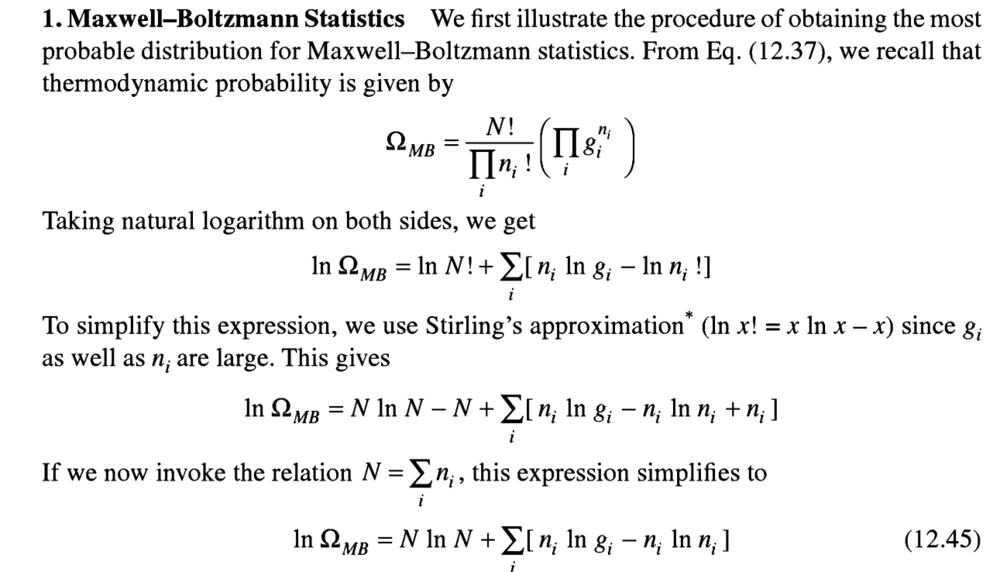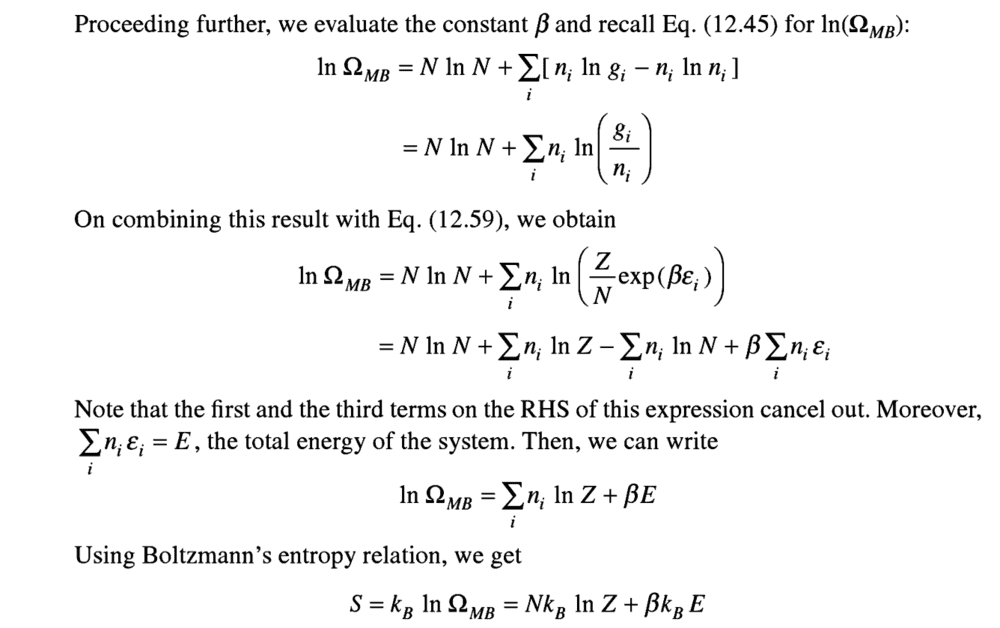# Deriving Sackur-Tetrode equation

• Kaguro

#### Kaguro

Homework Statement
Derive the Sackur-Tetrode equation.
Relevant Equations
...
Okay so I am learning Statistical mechanics from an Indian book "Thermal Physics,kinetic theory and statistical mechanics by Garg, Bansal and Ghosh".

I have derived the MB distribution function, and have evaluated the parameters α and β. With its help I derived the expression for entropy:

##S=k_B N ln(Z) + \frac{U}{T}##
Then we assumed that particles of an ideal monoatomic gas are distinguishable and derived the expression for its partition function:

##Z= V^N (\frac{mk_B T}{2 \pi \hbar^2})^{3N/2}##

So entropy becomes:

## S=Nk_B ( ln(V T^{3/2} )+ ln( \frac{mk_B e}{2\pi \hbar^2} ) )##
which tends to negative infinity as T tends to 0.

Also it gives rise to the Gibb's paradox.

To resolve it, Sackur suggested that we're over counting the microstates. So divide the thermodynamic probability W by N!.
Now, ##S=k_B ln(W)##

So, in the expression for entropy, there is another term: ##-k_B ln(N!)##
Now, ##S=k_B (N ln(Z) - ln(N!)) + \frac{U}{T}##

At this point the book says: "With this we can conclude that Boltzmann counting influences the partition function in the same way as it does thermodynamic probability. So ## Z^C = \frac{Z}{N!}## " ( Zc is Z corrected.)

How does it conclude that!?

Before: ##S=k_B N ln(Z) + \frac{U}{T}##
After: ##S=k_B (N ln(Z) - ln(N!)) + \frac{U}{T}##

For new Z to be Z/N! , there should have been an N multiplied with the ln(N!) and I could take it common.

##S=k_B N ln(Z) + \frac{U}{T}##
Are you sure that the factor of ##N## should be there in the first term on the right side?

Yes. Sorry there's so much that it'd take a lot of time to type it. Here's the derivation:This is how to get the expression for entropy.

The problem seems to be that in some of your equations, ##Z## represents the partition function of a single particle of the system, while in other equations ##Z## represents the partition function of the system of ##N## particles. If we let ##Z_1## denote the partition function of a single particle and ##Z_N## denote the partition function of the system, then for an ideal gas ##Z_N = Z_1^N## (without the Gibbs factor) and ##Z_N = Z_1^N/N!## (with the Gibbs factor.)

Note that if we are not including the Gibbs factor of ##1/N!##, then we have ##Nln(Z_1) = ln(Z_N)##.

When you wrote ##S=k_B N ln(Z) + \frac{U}{T}##, the ##Z## here should be ##Z_1##. This is before introducing the Gibbs factor. So, you can write this as

##S=k_B N ln(Z_1) + \frac{U}{T} = k_B ln(Z_N) + \frac{U}{T}##.

When you wrote ##Z= V^N (\frac{mk_B T}{2 \pi \hbar^2})^{3N/2}##, the ##Z## here is ##Z_N##.

The Gibbs correction factor is introduced for ##Z_N##, not ##Z_1##. So, your equation ## Z^C = \large \frac{Z}{N!}## is actually ## Z_N^C = \large \frac{Z_N}{N!}##

•vanhees71 and Kaguro
So this was it!

I now used the modified total partition function (without N multiplied outside) and I can now see that using Gibbs' factor in the thermodynamic probability W will lead to the equation for entropy looking as if I used the Gibbs' factor in the partition function. This is what the book claimed!

After this part rest is easy, just put in the partition function for ideal gas( the composite one) and obtain the S-T equation. This contains a V/N part so when I mix two same gases at the same density, there is no change in entropy. So Gibbs' paradox is resolved!

Thank you very much!

•TSny